Problem:

Write a program to check whether a given number is an ugly number.

Ugly numbers are positive numbers whose prime factors only include 2, 3, 5. For example, 6, 8 are ugly while 14 is not ugly since it includes another prime factor 7.

Note that 1 is typically treated as an ugly number.

Idea:

Math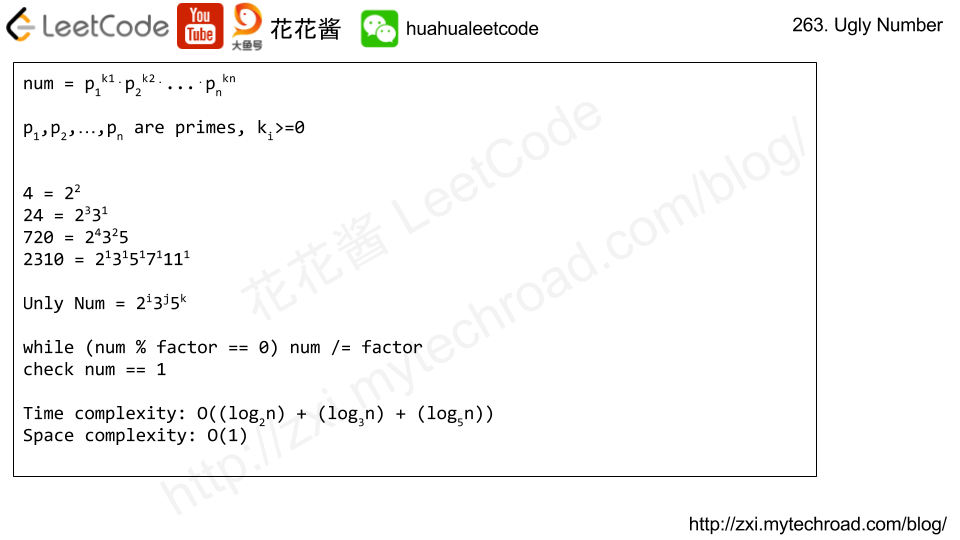Solution:

C++

Related Problems:

Given an array of integers that is already sorted in ascending order, find two numbers such that they add up to a specific target number.

The function twoSum should return indices of the two numbers such that they add up to the target, where index1 must be less than index2. Please note that your returned answers (both index1 and index2) are not zero-based.

You may assume that each input would have exactly one solution and you may not use the same element twice.

Input: numbers={2, 7, 11, 15}, target=9
Output: index1=1, index2=2

Solution:

C++ / two pointers

Time complexity: O(n)

Space complexity: O(1)

C++ / Binary search

Related Problems:

Problem:

We are given an elevation map, heights[i] representing the height of the terrain at that index. The width at each index is 1. After V units of water fall at index K, how much water is at each index?

Water first drops at index K and rests on top of the highest terrain or water at that index. Then, it flows according to the following rules:

• If the droplet would eventually fall by moving left, then move left.
• Otherwise, if the droplet would eventually fall by moving right, then move right.
• Otherwise, rise at it’s current position.

Here, “eventually fall” means that the droplet will eventually be at a lower level if it moves in that direction. Also, “level” means the height of the terrain plus any water in that column.

We can assume there’s infinitely high terrain on the two sides out of bounds of the array. Also, there could not be partial water being spread out evenly on more than 1 grid block – each unit of water has to be in exactly one block.

Idea: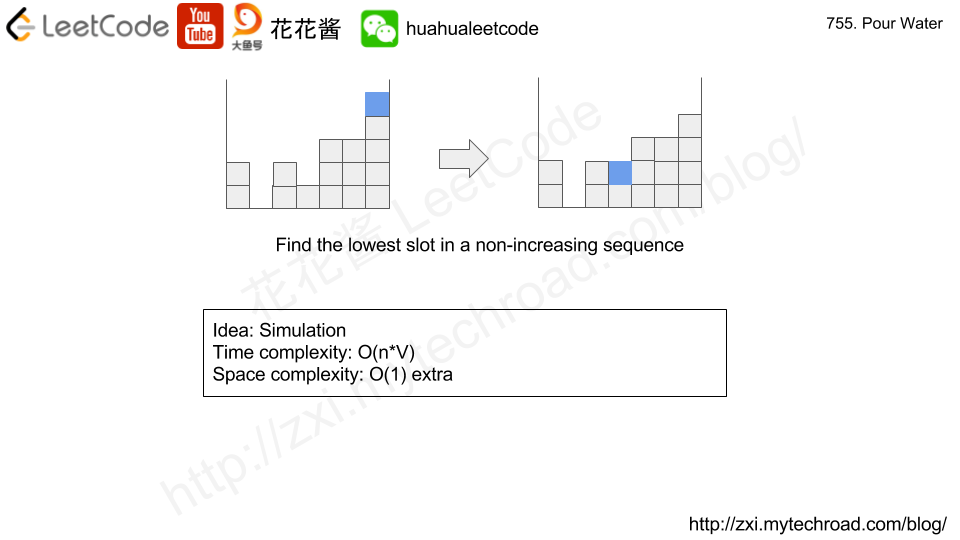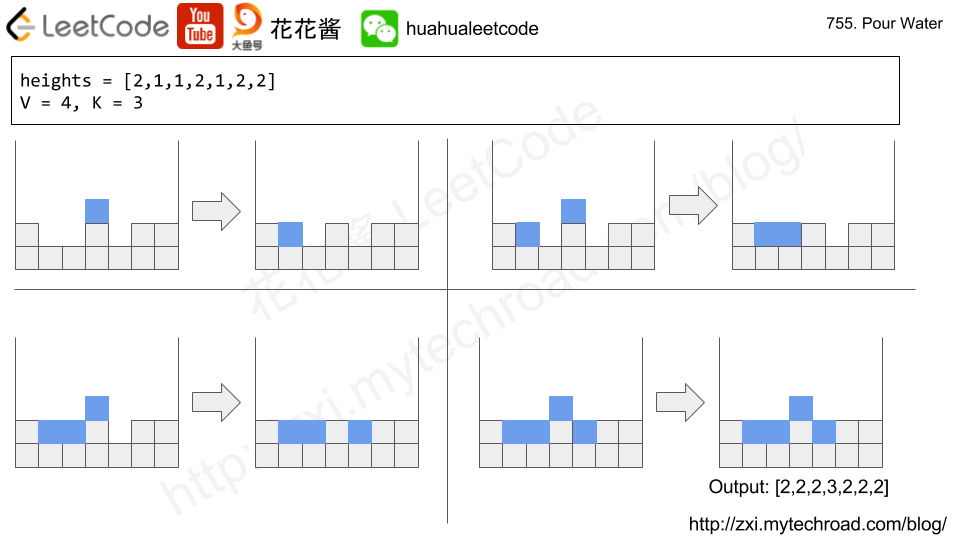# Solution 1: Simulation

Time complexity: O(V*n)

Space complexity: O(1)

## Python3

Say you have an array for which the ith element is the price of a given stock on day i.

Design an algorithm to find the maximum profit. You may complete as many transactions as you like (ie, buy one and sell one share of the stock multiple times) with the following restrictions:

• You may not engage in multiple transactions at the same time (ie, you must sell the stock before you buy again).
• After you sell your stock, you cannot buy stock on next day. (ie, cooldown 1 day)

Example:

Idea:

DP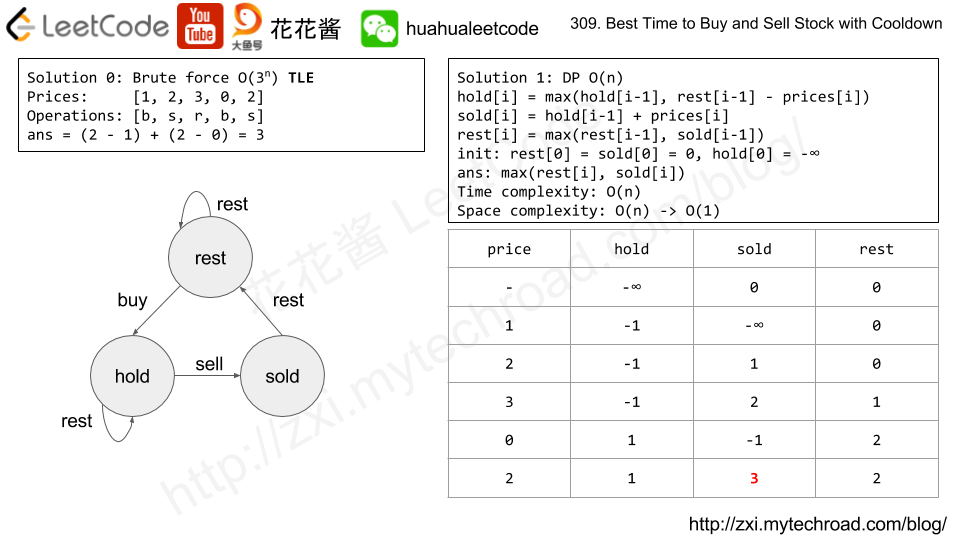Solution:

Time complexity: O(n)

Space complexity: O(1)

C++

Related Problems:

In this episode, we will introduce Fenwick Tree/Binary Indexed Tree, its idea and implementation and show its applications in leetcode.

Fenwick Tree is mainly designed for solving the single point update range sum problems. e.g. what’s the sum between i-th and j-th element while the values of the elements are mutable.

Init the tree (include building all prefix sums) takes O(nlogn)

Update the value of an element takes O(logn)

Query the range sum takes O(logn)

Space complexity: O(n)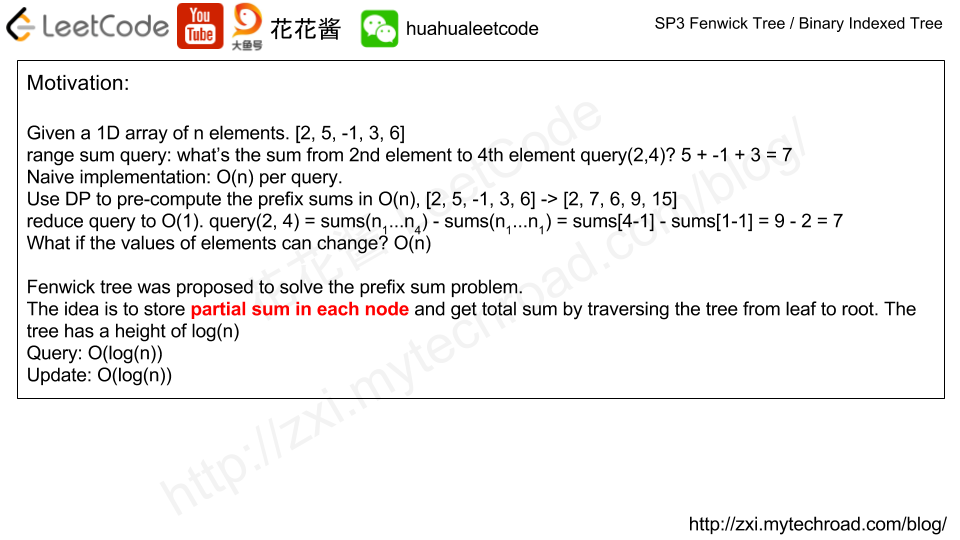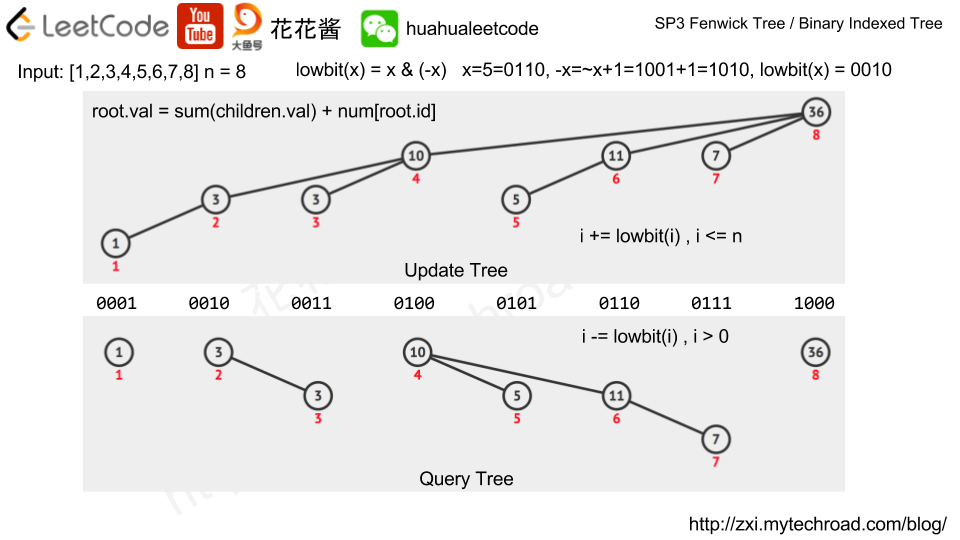Applications:

Implementation:

## Python3

Mission News Theme by Compete Themes.